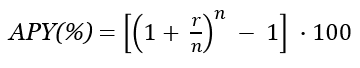# APY Calculator

Use this online APY calculator to easily calculate the APY (Annual Percentage Yield) of a deposit based on the simple annual interest rate and the compounding period. Allows calculating APY of savings based on daily, monthly, quarterly, semiannual, and annual interest compounding, corresponding to compounding once per day, month, quarter, 6-months and 12-months (once per year).

%
\$
Share calculator:

Embed this tool:
get code

## Using the APY calculator

Compound interest is a type of interest in which the interest amount is periodically added to the principal amount and new interest is subsequently accrued over interest from past periods. While some financial institutions will list the annual percentage yield (APY), a.k.a. effective interest rate of their products, many only list the annual percentage rate (APR) which is a simple multiplication and doesn't reflect your actual interest rate. In such cases it is best to calculate the annual percentage yield, so you know how much your Certificate of Deposit will actually generate for you.

Our online APY calculator is a versatile tool which calculates:

• the annual percentage yield / effective interest rate of a deposit
• the final amount of money you will be able to save if you specify starting capital and deposit term

Start by entering the simple annual interest rate (ARP) and continue by specifying the compounding period - you may need to consult your bank on this detail, but it is often disclosed on offers and deposit descriptions. Finally, if you plan on making regular contributions: adding to the deposit on a regular basis (monthly, yearly, etc.), enter the amount and the period on which you will make it, as well as whether you will make it in the beginning or the end of the period.

Optionally, you can enter the starting balance of your deposit as well as its term, which will allow our tool to calculate the total capital growth and earnings from interest you can expect.

Our interest calculator will output: the Annual Percentage Yield (APY), the value of your deposit or investment at the end of the period, the interest accrued, and the percentage capital growth. Note that none of these calculation takes into account bank fees, taxes, servicing fees, etc. which may apply.

## APY formula

If you wonder how to calculate the APY by yourself or using an Excel spreadsheet, all you need is the annual percentage yield formula:where r is the simple annual interest rate in decimal, n is the number of compounding periods per year. For example, with an annual interest rate on a Certificate of Deposit of 2% and quarterly compounding, the calculation is APY = ((1 + 0.02/4)4 - 1) * 100 = ((1.020154) - 1) * 100 = (1.02015 - 1) * 100 = 2.015% annual percentage yield.

## Effect of the compounding frequency

The compounding frequency, which is the time period at which interest is added to the principal, can have a slight positive effect on the effective interest rate of a Certificate of Deposit (CD) versus the nominal annual interest rate. Entering shorter compounding periods in the above CD calculator will easily show you how big that effect could be on your overall savings. You get the best effective rate when you have daily compounding (daily interest, also called continuous compounding) and slightly worse with monthly or yearly compounding.

Comparison of Annual Percentage Yield of CDs with different compounding periods
Initial DepositAnnual Interest RateCompounding FrequencyAPYValue after 5y
\$10,000 3% Annually 3.000% \$11,593
\$10,000 3% Semiannually 3.022% \$11,605
\$10,000 3% Quarterly 3.034% \$11,612
\$10,000 3% Monthly 3.042% \$11,616
\$10,000 3% Daily 3.045% \$11,618

The above assumes no additional deposits to simplify the comparison. As you can see for small sums the APY is quite close to the annual interest rate and makes little difference, especially over short periods of time. This effect is larger with longer time periods and becomes substantial for large deposit amounts.

## Financial caution

This is a simple calculator which is a good starting point in estimating the interest rate and returns from a bank deposit or a similar investment, but is by no means the end of such a process. You should always consult a qualified professional when making important financial decisions and long-term agreements, such as long-term bank deposits. Use the information provided by this software critically and at your own risk.

#### Cite this calculator & page

If you'd like to cite this online calculator resource and information as provided on the page, you can use the following citation:
Georgiev G.Z., "APY Calculator", [online] Available at: https://www.gigacalculator.com/calculators/apy-calculator.php URL [Accessed Date: 28 Sep, 2023].